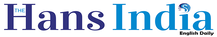# Ways to select stocks using moving averagesx
Highlights

## Generally, each trader has a different trading strategies. One can make profits with different strategies but a repeated methodology will give consistent profits.

Generally, each trader has a different trading strategies. One can make profits with different strategies but a repeated methodology will give consistent profits.

Usually, a trader tries one strategy and if it doesn't work, he shifts to another but one should remember that repetition is the key to success. The most popular method is that of moving averages.

Moving averages are of two types - simple and exponential. Exponential is mostly preferred by traders as it reacts more quickly to change in price. Suppose a 21-day moving average is plotted on a chart, it suggests possible entry levels.

Whenever the price touches the moving average or even if it crosses, we are not supposed to take an entry. If it consolidates after crossing the moving average, then it makes a clear upward move. That would be an ideal level to make an entry to earn sure shot profit.

An ideal timeframe for this kind of a setup would be a15 minute timeframe. The trader would take the trade only when it has consolidated on the 21-day exponential moving average.

Consolidation in other words is where the price would trade in a range-bound area for a while. In such, a situation the price would be moving in a rectangular area.

Suppose we are in a 15-minute timeframe with 21-day EMA, then we need to see price consolidation at least in four 15-minute candles. If we take a trade without consolidation, then we might lose capital by making huge losses. In such trades, accuracy would be around 85 percent.

Generally, when we trade in moving averages, crossover system is used. In crossover strategy, two moving averages are plotted. For example, if we plot a 8/13 day moving average, we take a trade whenever there is a crossover.

But in the particular strategy which we are discussing, we simply plot a 21-day moving average and initiate a trade. When the moving average curve cuts the price from below, we initiate a buy trade.

If the moving average cuts the price from above, we initiate a short sell trade. In this strategy on a 5-minute chart, we may get around 4 trades and on a 10 minutes timeframe we may get 2 or three trades.

A fifteen minute would give only one or 2 trades. As the timeframe increases, the number of entries islimited. Even though moving average is a lagging indicator, it by and large generates accurate trade.

(The author is a homemaker who dabbles in stock market investments in free time)

More On
Interested in blogging for thehansindia.com? We will be happy to have you on board as a blogger.Next Story
More Stories